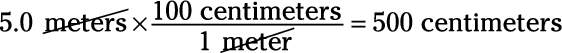Connect with us
Course Content
Unit Conversion
What is Unit Conversion? Unit conversion is a process with multiple steps that involves multiplication or division by a numerical factor or, particularly a conversion factor. The process may also require selection of the correct number of significant digits, and rounding. Different units of conversion are used to measure different parameters.
0/5
Unit Conversion in Stochiometry
0/2
Unit Conversion

# Unit Conversion

• Length:

• 1 m = 100 cm

• 1 km = 1,000 m

• 1 in (inch) = 2.54 cm

• 1 m = 39.37 in

• 1 mile = 5,280 ft = 1.609 km

• 1 angstrom = 10–10 m

• 1 ft = 12 in

• 3ft = 1 yard
• ### Area Unit Conversions

 1 sq. inch 6.4516×10-4 sq. meters 1 sq. foot 9.2903×10-2 sq. meters 1 acre 4.0468×103 sq. meters 1 hectare 1×104 sq. meters 1 sq. mile 2.5888×106 sq. meters 1 barn 1×10-28 square meters
• Volume:

• 1 l (liter) = 1000 ml

• 1ml (milliliter) = 1 cm3

• 1m3 = 1000l

• 1 gallon = 3.785l

• 1 quart = 2 pints
• Mass:

• 1 kg = 1,000 g = 2.21 pounds

• 1 slug = 14.59 kg

• 1 u (atomic mass unit) = 1.6605 x 10–27 kg

• Force:

• 1 lb (pound) = 4.448 N = 160 ounces

• 1 ton = 2000 pounds

• 1 metric ton = 1000kg = 2204 pounds
• 1 N = 105 dynes

• 1 N = 0.2248 lb

• Energy:

• 1 J = 107 ergs

• 1 J = 0.7376 ft-lb

• 1 BTU (British thermal unit) = 1,055 J

• 1 kWh (kilowatt hour) = 3.600 x 106 J

• 1 eV (electron volt) = 1.602 x 10–19 J

• Power:

• 1 hp (horsepower) = 550 ft-lb/s

• 1 W (watt) = 0.7376 ft-lb/s

• Other Unit Conversions
• Yotta = 1024
• Zetta = 1021
• Exa = 1018
• Peta = 1015
• Tera = 1012
• Giga = 109
• Mega = 106
• Kilo = 103
• Hecto = 102
• Deca = 101
•
•
• Yecto = 10-24
• Zepto = 10-21
• Atto = 10-18
• Fecto = 10-15
• Pico = 10-12
• Nano = 10-9
• Micro = 10-6
• Milli = 10-3
• Centi = 10-2
• Deci = 10-1

Because conversions are such an important part of physics problems, and because you have to keep track of them so carefully, there’s a systematic way of handling conversions: You multiply by a conversion constant that equals 1, such that the units you don’t want cancel out.

## Sample question

1. A ball drops 5 meters. How many centimeters did it drop?

The correct answer is 500 centimeters. To perform the conversion, you do the following calculation:Note that 100 centimeters divided by 1 meter equals 1 because there are 100 centimeters in a meter. In the calculation, the units you don’t want — meters — cancel out.